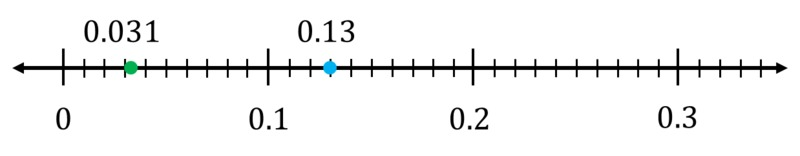# Comparing Decimals on the Number Line

Alignments to Content Standards: 5.NBT.A.3

1. Which is greater, 0.1 or 0.01? Show the comparison on the number line.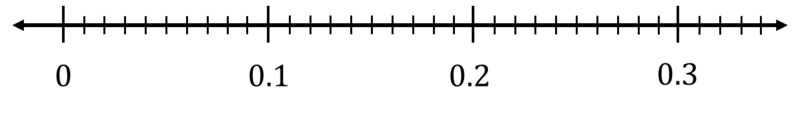2. Which is greater, 0.2 or 0.03? Show the comparison on the number line.3. Which is greater, 0.12 or 0.21? Show the comparison on the number line.4. Which is greater, 0.13 or 0.031? Show the comparison on the number line.## IM Commentary

This task involves using number lines to compare decimal numbers. The numbers selected in this task are purposefully chosen to target student misconceptions. For example, when asked to compare 0.13 and 0.031, students who are not yet proficient in decimal applications of our base ten system may rush to say that 0.031 is greater than 0.13 because 31 is greater than 13. This task also challenges students to think carefully about the value of each digit and how that affects the number's placement on the number line. Students are often presented with number lines that allow them to plot each number on a tick mark. In the last part of this task, students will grapple with placing a number between tick marks. It is important for students to be able to visualize the approximate location of numbers on the number line. A good follow-up task for this one would be 5.NBT Placing Thousandths on the Number Line. Notice that the language in the solutions underscores that the greater number will always be farther right on the number line. This reasoning should be heavily emphasized in discussion as it will transfer across comparisons of all rational numbers.

## Solution

1. $0.1 \gt 0.01$ because 0.1 is to the right of 0.01 on the number line.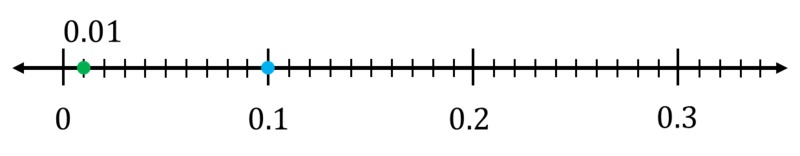2. $0.2 \gt 0.03$ because 0.2 is to the right of 0.03 on the number line.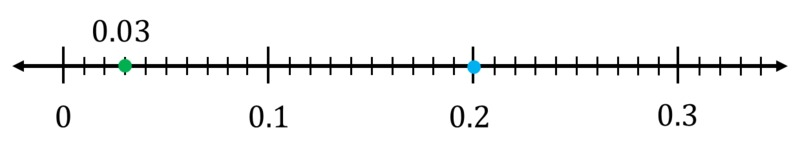3. $0.21 \gt 0.12$ because 0.21 is to the right of 0.12 on the number line.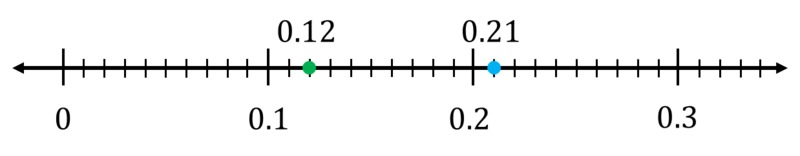4. $0.13 \gt 0.031$ because 0.13 is to the right of 0.031 on the number line.